## a spring of spring constant k=12.5N/m is hung vertically. a 0.500kg mass is then suspended from the spring. what is the displacement of the

Question

a spring of spring constant k=12.5N/m is hung vertically. a 0.500kg mass is then suspended from the spring. what is the displacement of the end of the spring due to the wieght of the 0.500 kg mass?

in progress 0
5 months 2021-08-18T01:32:50+00:00 1 Answers 39 views 0

The displacement of one end of the spring due to weight = 0.4 m.

Explanation:

Given :

Spring constant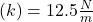Mass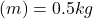From the hooke’s law,

Force is proportional to the negative of the displacement.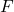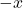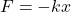Where minus sign represent that, the force is always directed toward mean position.

Now one force is gravity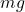acts as a downward. so we write,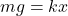Here we take only magnitude.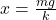Where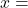displacement of one end of spring,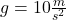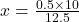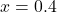m